# 模型

## 定义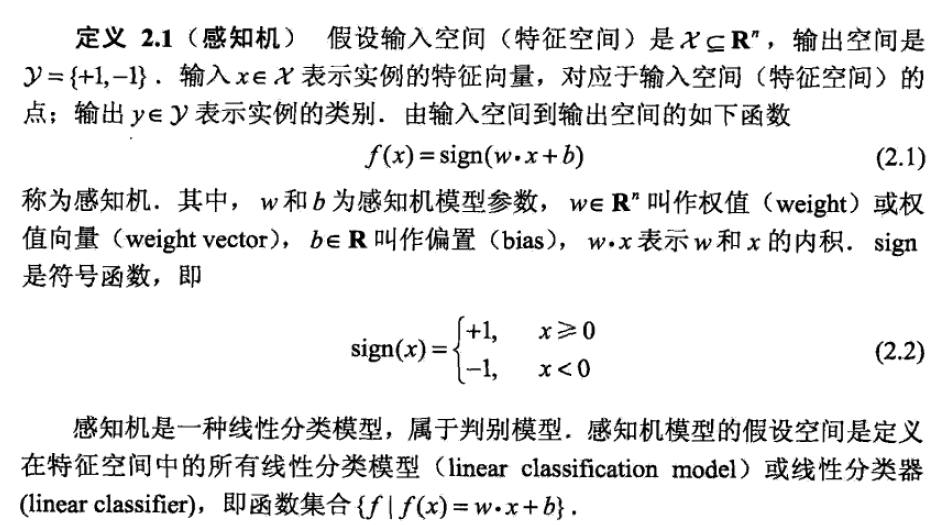## 几何学解释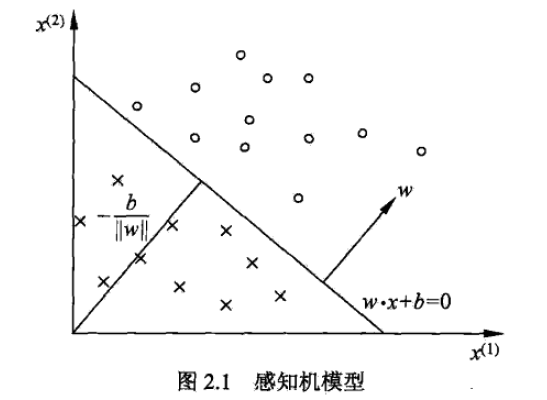# 学习策略

## 线性可分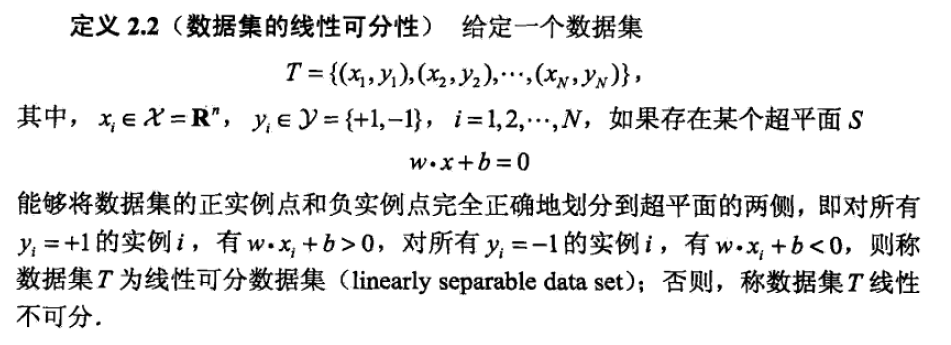## 损失函数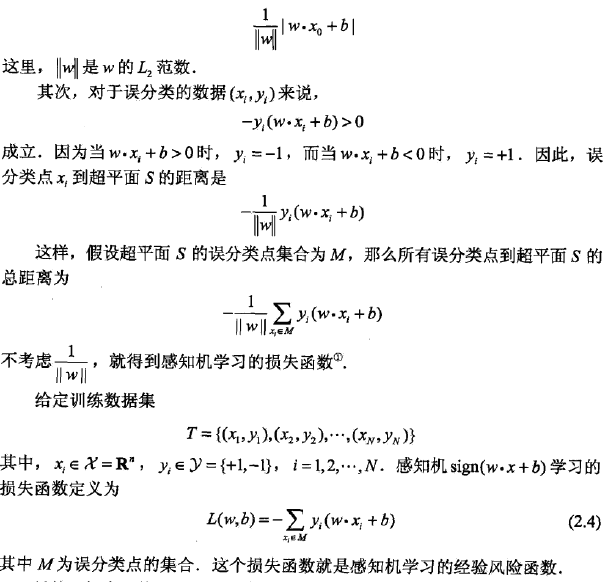# 学习算法

## 基础算法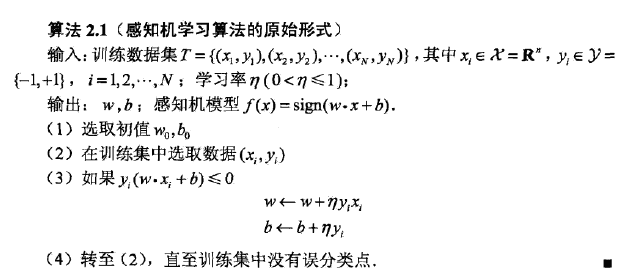## 代码实现

import pandas as pd
import numpy as np
import matplotlib.pyplot as plt

x = np.array([[3,3], [4,3], [1,1]])
y = [1, 1, -1]

w = [0 ,0]
b = 0
yita = 1

# 是否还存在误分类点
def isHasMisclassification(x, y, w, b):
misclassification = False
ct = 0
misclassification_index = 0
for i in range(0, len(y)):
if y[i]*(np.dot(w, x[i]) + b) <= 0:
ct += 1
misclassification_index = i
break
if ct>0:
misclassification = True
return misclassification, misclassification_index

# 更新系数w, b
def update(x, y, w, b, i):
w = w + y[i]*x[i]
b = b + y[i]
return w, b

# 更新迭代
def optimization(x, y, w, b):
misclassification, misclassification_index = isHasMisclassification(x, y, w, b)
while misclassification:
print ("误分类的点：", misclassification_index)
w, b = update(x, y, w, b, misclassification_index)
print ("采用误分类点 {} 更新后的权重为:w是 {} , b是 {} ".format(misclassification_index, w, b))
misclassification, misclassification_index = isHasMisclassification(x, y, w, b)
return w, b

w, b = optimization(x, y, w, b)

w,b


误分类的点： 0

(array([1, 1]), -3)


# 对偶形式

## 对偶算法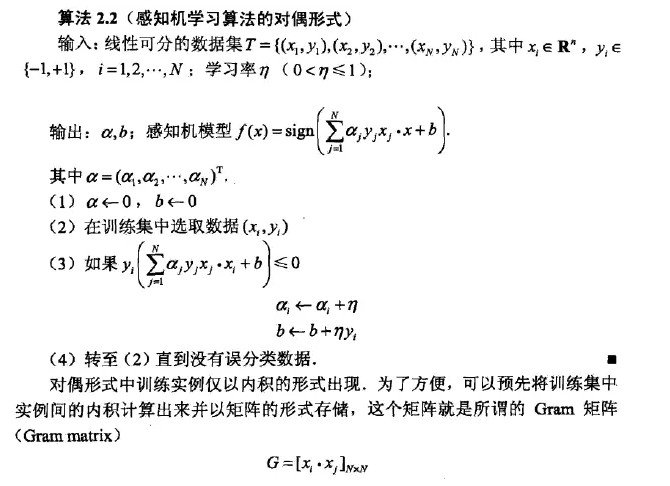alpha_i 标识第 i 个实例点因为误分而进行的更新的次数。

## 理解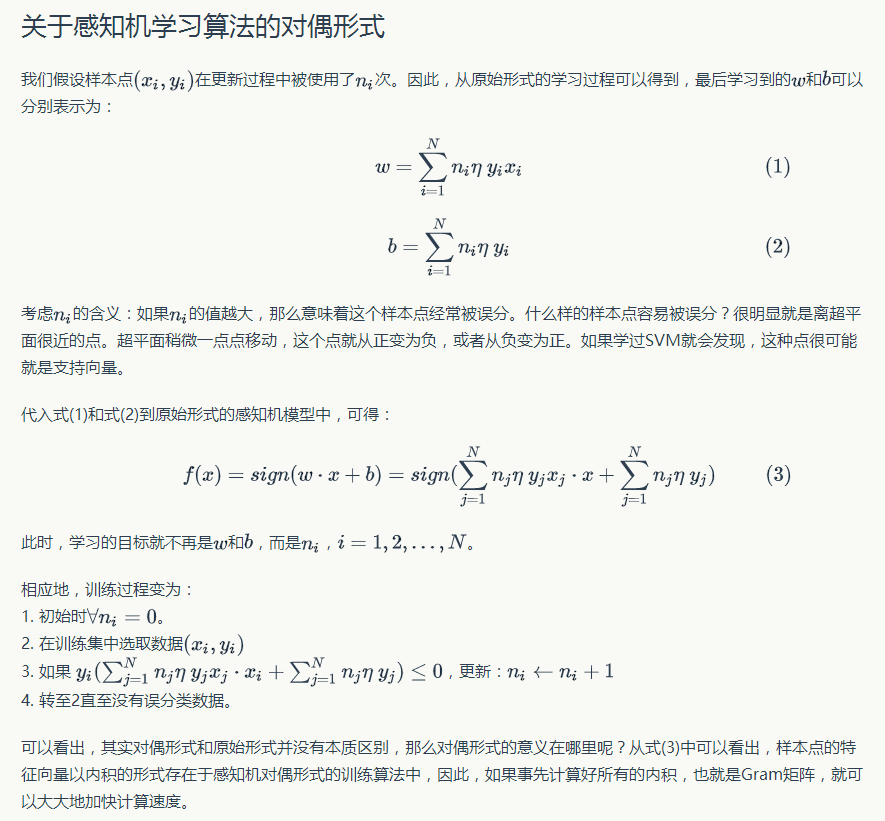## 算法实现

import pandas as pd
import numpy as np
import matplotlib.pyplot as plt

x = np.array([[3,3], [4,3], [1,1]])
x_transpose = x.T
y = [1, 1, -1]
g = np.dot(x, x_transpose)   #gram 矩阵

alfa = np.array([0, 0, 0])   # alpha i
b = 0   #截距
yita = 1

#是否还存在误分类点
def isHasMisclassification(y, g, b):
misclassification = False
ct = 0
misclassification_index = 0
for i in range(0, len(y)):
sum1 = 0
for j in range(0, len(y)):
sum1 += (alfa[j]*y[j]*g[j][i] + b)
if y[i]*sum1 <= 0:
ct += 1
misclassification_index = i
break
if ct > 0:
misclassification = True
return misclassification, misclassification_index

# 更新系数alfa, b
def update(y, alfa, yita, b, i):
alfa[i] = alfa[i] + yita
b = b + yita*y[i]
return alfa, b

# 更新迭代
def optimization(y, alfa, b, yita):
misclassification, misclassification_index = isHasMisclassification(y, g, b)
while misclassification:
print ("误分类的第{}点{}：".format(misclassification_index, x[misclassification_index]))
alfa, b = update(y, alfa, yita, b, misclassification_index)
print ("采用第{}误分类点 {} 更新后的权重为:alfa是 {} , b是 {} ".format(misclassification_index, x[misclassification_index], alfa, b))
misclassification, misclassification_index = isHasMisclassification(y, g, b)
return alfa, b

alfa, b = optimization(y, alfa, b, yita)

# In:
#w=sum(alfa_i*y_i*x_i)
alfa_y = np.multiply(list(alfa),y)
w = np.dot(alfa_y,x)
b = np.dot(alfa, y)

w,b


[[18 21  6]
[21 25  7]
[ 6  7  2]]


# 参考资料

《统计学习方法》

【统计学习方法.李航】感知机学习算法